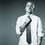# A problem related to Vectors.

Hi, I'm new here. Please excuse me if I break some rule of this website.

Three particles $$A,B$$ and $$C$$ are at the vertices of an equilateral trianagle $$ABC$$. Each of the particle moves with constant speed $$v$$. $$A$$ always has its velocity along $$AB$$, $$B$$ along $$BC$$ and $$C$$ along $$CA$$. They meet each other at the centroid. At any instant, the component of velocity of B along BA is $$v\cos60^∘$$.

I know that by symmetry $ABC$ will always make an equilateral triangle. But can we prove this mathematically, that is

• Can we mathematically show that at any instant of time $t$ the points $A(t),B(t),C(t)$ will make an equilateral triangle whose centroid is the same as that of the triangle made by the points $A(0),B(0),C(0)$?

My own understanding:
Since the all the distances $AB,BC$ and $CA$ decreases at the same rate the points $ABC$ must meet coincidentally and at any instant $ABC$ should make a equilateral triangle. Now I have to prove that the centroid must remain constant. For this I am thinking of two methods
(1) I choose the initial centroid as the origin and label the points $A,B$ and $C$ as $\vec r_A,\vec r_B, \vec r_C$ respectively now $|\vec r_A(0)|=|\vec r_B(0)|=|\vec r_C(0)|$ and $\dfrac {d|(\vec r_B- \vec r_A)|}{dt}=const$...

(2) For simplification I choose the point $A$ as the centre of the coordinate system and.....

As the things were going tedious I thought it would be better to ask.Note by Anupam Khosla
6 years, 1 month ago

This discussion board is a place to discuss our Daily Challenges and the math and science related to those challenges. Explanations are more than just a solution — they should explain the steps and thinking strategies that you used to obtain the solution. Comments should further the discussion of math and science.

When posting on Brilliant:

• Use the emojis to react to an explanation, whether you're congratulating a job well done , or just really confused .
• Ask specific questions about the challenge or the steps in somebody's explanation. Well-posed questions can add a lot to the discussion, but posting "I don't understand!" doesn't help anyone.
• Try to contribute something new to the discussion, whether it is an extension, generalization or other idea related to the challenge.

MarkdownAppears as
*italics* or _italics_ italics
**bold** or __bold__ bold
- bulleted- list
• bulleted
• list
1. numbered2. list
1. numbered
2. list
Note: you must add a full line of space before and after lists for them to show up correctly
paragraph 1paragraph 2

paragraph 1

paragraph 2

[example link](https://brilliant.org)example link
> This is a quote
This is a quote
    # I indented these lines
# 4 spaces, and now they show
# up as a code block.

print "hello world"
# I indented these lines
# 4 spaces, and now they show
# up as a code block.

print "hello world"
MathAppears as
Remember to wrap math in $$ ... $$ or $ ... $ to ensure proper formatting.
2 \times 3 $2 \times 3$
2^{34} $2^{34}$
a_{i-1} $a_{i-1}$
\frac{2}{3} $\frac{2}{3}$
\sqrt{2} $\sqrt{2}$
\sum_{i=1}^3 $\sum_{i=1}^3$
\sin \theta $\sin \theta$
\boxed{123} $\boxed{123}$

Sort by:

what if the triangle is scalene???

- 6 years ago

It resembles a cyclic movement.... position of vertices change but the centre point remains same.... looks like as if the triangle is rotating..

- 6 years, 1 month ago

A solved example of Concepts of Physics by H.C.Verma

- 6 years, 1 month ago

yes we can simply prove it.take some value of the sides of the equilateral triangle.now we know the velocities each of them and again we know their directions,so now we can calculate how much time it will take to meet each other.now take a time less than that,and get the co -ordinates of position of that time,now take a suitable co-ordinate system of your own and place the value then simply you will prove that you want to.

- 6 years, 1 month ago

Look, try to picture this in your head. If we have an equilateral triangle, and we try to imagine that all the sides decreases at an equal and uniform rate, its center of gravity will be unchanged.

If you are confused, just think about the fact that if the rates at which the sides change are not equal, then it will no longer be an equilateral triangle. Then the centroid WILL CHANGE.

I hope this helps.

- 6 years, 1 month ago

I think I got it. Since all the sides decreases at the same rate so it should always be an equilateral triangle. Thankyou

- 6 years, 1 month ago

I dont know what you're doing, I just know you said all particles move cyclically towards one another [A to B, B to C & C to A] in an equilateral right? thereby the sum of their velocities at every instant is zero..., no collision, no interaction, nothing... therefore momentum conservation reveals centre of mass remains at same location..., to correlate centre of mass with centroid I assume m1=m2=m3, which says that Centre of mass, or the centroid, wont move.

- 6 years, 1 month ago

It looks likes that you are a "shady"fan & are you really 106 !

- 6 years, 1 month ago

Do you know how to edit "age" in the profile?

- 6 years, 1 month ago

No, I'm 20. Haven't got familiar with this website yet.

- 6 years, 1 month ago